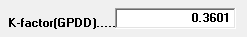Static K Routing Method

# Static K Routing Method

This method is very similar to the Degree Day routing method, except the K Factor does not change.

If you're unfamiliar with Degree Days, see Overview of Degree Days.
If you're unfamiliar with the Degree Day routing method, see Degree Day Routing Method.

How it works: Go to the Routing window for the tank and select the "Degree Days" routing method. Then enter the K-factor for this tank in thetext box. The system will use this factor to calculate return dates. For an explanation of K-factors, left-click here.

### Static K and Gallons Per Day

If you're unfamiliar with the Gallons per Day routing method, see Gallons Per Day Routing Method.

How it works: This routing method uses a combination of both the Gallons Per Day and Static K-Factor to determine the next delivery date. For instance, this might be used if a customer has both a water heater (where usage is consistent and Gallons Per Day is the best way to estimate usage) and a heating appliance (where Degree Days is more appropriate).